## Laplacian

The Laplacian operator for a Scalar functionis defined by(1)

in Vector notation, where the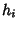are the Scale Factors of the coordinate system. In Tensor notation, the Laplacian is written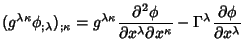(2)

whereis a Covariant Derivative and(3)

The finite difference form is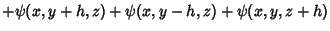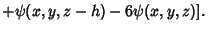(4)
For a pure radial function,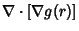(5)

Using the Vector Derivative identity(6)

so(7)

Therefore, for a radial Power law,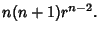(8)

A Vector Laplacian can also be defined for a Vector A by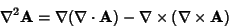(9)

in vector notation. In tensor notation, A is written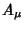, and the identity becomes(10)

Similarly, a Tensor Laplacian can be given by(11)

An identity satisfied by the Laplacian is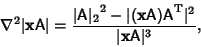(12)

whereis the Hilbert-Schmidt Norm,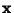is a row Vector, and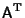is the Matrix Transpose of A.

To compute the Laplacian of the inverse distance function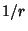, where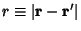, and integrate the Laplacian over a volume,(13)

This is equal to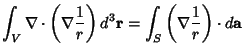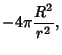(14)

where the integration is over a small Sphere of Radius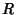. Now, forand, the integral becomes 0. Similarly, for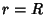and, the integral becomes. Therefore,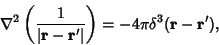(15)

whereis the Delta Function.